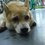# Ideal solution ~ Raoult's Law

Definition of Ideal solution : In chemistry, an ideal solution or ideal mixture is a solution with thermodynamic properties analogous to those of a mixture of ideal gases

Definition : Raoult's law states that the vapor pressure of a solvent above a solution is equal to the vapor pressure of the pure solvent at the same temperature scaled by the mole fraction of the solvent present.

Introduction : In the 1880s, French chemist François-Marie Raoult discovered that when a substance is dissolved in a solution, the vapor pressure of the solution will generally decrease. This observation depends on two variables:

(1) the mole fraction of the amount of dissolved solute present

(2)* the original vapor pressure*

$P_{solution}$= $X_{solvent}$ x $P_{solvent}^0$

At any given temperature for a particular solid or liquid, there is a pressure at which the vapor formed above the substance is in dynamic equilibrium with its liquid or solid form. This is the vapor pressure of the substance at that temperature. At equilibrium, the rate at which the solid or liquid evaporates is equal to the rate that the gas is condensing back to its original form. All solids and liquids have vapor pressure, and this pressure is constant regardless of how much of the substance is present.

Ideal vs. Nonideal Solutions

Raoult's Law only works for ideal solutions. An ideal solution is defined as one which obeys Raoult's Law. "An ideal solution shows thermodynamic mixing characteristics identical to those of ideal gas mixtures [except] ideal solutions have intermolecular interactions equal to those of the pure components."

Like many other concepts explored in Chemistry, Raoult's Law only applies under ideal conditions in an ideal solution. However, it still works fairly well for the solvent in dilute solutions. In reality though, the decrease in vapor pressure will be greater than that calculated by Raoult's Law for extremely dilute solutions.Note by Nicole Ling
7 years, 3 months ago

This discussion board is a place to discuss our Daily Challenges and the math and science related to those challenges. Explanations are more than just a solution — they should explain the steps and thinking strategies that you used to obtain the solution. Comments should further the discussion of math and science.

When posting on Brilliant:

• Use the emojis to react to an explanation, whether you're congratulating a job well done , or just really confused .
• Ask specific questions about the challenge or the steps in somebody's explanation. Well-posed questions can add a lot to the discussion, but posting "I don't understand!" doesn't help anyone.
• Try to contribute something new to the discussion, whether it is an extension, generalization or other idea related to the challenge.
• Stay on topic — we're all here to learn more about math and science, not to hear about your favorite get-rich-quick scheme or current world events.

MarkdownAppears as
*italics* or _italics_ italics
**bold** or __bold__ bold
- bulleted- list
• bulleted
• list
1. numbered2. list
1. numbered
2. list
Note: you must add a full line of space before and after lists for them to show up correctly
paragraph 1paragraph 2

paragraph 1

paragraph 2

[example link](https://brilliant.org)example link
> This is a quote
This is a quote
    # I indented these lines
# 4 spaces, and now they show
# up as a code block.

print "hello world"
# I indented these lines
# 4 spaces, and now they show
# up as a code block.

print "hello world"
MathAppears as
Remember to wrap math in $$ ... $$ or $ ... $ to ensure proper formatting.
2 \times 3 $2 \times 3$
2^{34} $2^{34}$
a_{i-1} $a_{i-1}$
\frac{2}{3} $\frac{2}{3}$
\sqrt{2} $\sqrt{2}$
\sum_{i=1}^3 $\sum_{i=1}^3$
\sin \theta $\sin \theta$
\boxed{123} $\boxed{123}$

## Comments

There are no comments in this discussion.

×

Problem Loading...

Note Loading...

Set Loading...